# HSSlive: Plus One & Plus Two Notes & Solutions for Kerala State Board

## Tuesday, June 28, 2022BSEB Class 9 Maths Chapter 8 Quadrilaterals Ex 8.2 Textbook Solutions PDF: Download Bihar Board STD 9th Maths Chapter 8 Quadrilaterals Ex 8.2 Book Answers

## Bihar Board Class 9th Maths Chapter 8 Quadrilaterals Ex 8.2 Books Solutions

 Board BSEB Materials Textbook Solutions/Guide Format DOC/PDF Class 9th Subject Maths Chapter 8 Quadrilaterals Ex 8.2 Chapters All Provider Hsslive

2. Click on the Bihar Board Class 9th Maths Chapter 8 Quadrilaterals Ex 8.2 Answers.
3. Look for your Bihar Board STD 9th Maths Chapter 8 Quadrilaterals Ex 8.2 Textbooks PDF.

Find below the list of all BSEB Class 9th Maths Chapter 8 Quadrilaterals Ex 8.2 Textbook Solutions for PDF’s for you to download and prepare for the upcoming exams:

## BSEB Bihar Board Class 9th Maths Solutions Chapter 8 Quadrilaterals Ex 8.2

Question 1.
ABCD is a quadrilateral in which P, Q, R and S are mid-points of the sides AB, BC, CD and DA (see figure). AC is a diagonal. Show that :(i) SR || AC and SR = 12 AC
(ii) PQ = SR
(iii) PQRS is a parallelogram.
Solution:
Given : A quadrilateral ABCD in which P, Q, R and S are respectively the mid-points of the sides AB, BC, CD and DA. Also AC is its diagonal.
To prove : (i) SR || AC and SR = 12 AC
(ii) PQ = SR
(iii) PQRS is a parallelogram.
Proof :
(i) In Δ ACD, we have
S is the mid-point of AD and R is the mid-point of CD. Then SR || AC and SR = 12AC [Mid-point theorem]

(ii) In Δ ABC, we have
P is the mid-point of the side AB and Q is the mid-point of the side BC.
Then PQ || AC
and PQ = 12AC
Thus, we have proved that: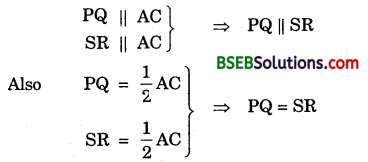(iii) Since PQ = SR and PQ || SR
⇒ One pair of opposite sides are equal and parallel.
⇒ PQRS is a parallelogram.

Question 2.
ABCD is a rhombus and P, Q, R and S are respectively the mid-points of the sides AB, BC, CD and DA respectively. Show that the quadrilateral PQRS is a rectangle.
Solution:
Given : ABCD is a rhombus in which P, Q, R and S are the mid-points of AB, BC, CD and DA respectively. PQ, QR, RS and SP are joined to obtain a quadrilateral PQRS.To prove : PQRS is a rectangle.
Construction : Join AC.
Proof:
In Δ ABC, P and Q are the mid-points of AB and
∴ PQ || AC and PQ = 12AC … (1)
Similarly, in A ADC, R and S are the mid-points of CD and PD.
∴ SR || AC and SR = 12AC
From (1) and (2), we get
PQ || RS and PQ = SR
Now, in quad. PQRS its one pair of opposite sides PQ and SR is equal and parallel.
∴ PQRS is a parallelogram
∴ AB = BC [Sides of a rhombus]
⇒ 12AB = 12BC
⇒ PB = BQ
⇒ ∠3 = ∠4 [∠s opp. to equal sides of a A]
Now, in ∆ APS and ∆ CQR, we have
AP = CQ [Halves of equal sides AB, BC]
AS = CR [Halves of equal sides AD, CD]
PS = QR [Opp. sides of parallelogram PQRS]
∴ ∆ APS = ∆ CQR [SSS Cong. Theorem]
⇒ ∠1 = ∠2 [C.P.C.T.]
Now, ∠1 + ∠SPQ + ∠3 = 180° [Linear pair axiom]
∴ ∠1 + ∠PQ + ∠3 = ∠2 + ∠PQR + ∠4
But ∠1 = ∠2 and ∠3 = ∠4[Proved above]
∴ ∠SPQ = ∠PQR … (3)
∴ SP || RQ intersects them,
∴ ∠SPQ + ∠PQR = 180° … (4)
From (3) and (4), we get
∠SPQ = ∠PQR = 90°
Thus, PQRS is a parallelogram whose one angle ∠SPQ = 90°
Hence, PQRS is a rectangle.

Question 3.
ABCD is a rectangle and P, Q, R and S are mid¬points of the sides AB, BC, CD and DA respectively. Show that the quadrilateral PQRS is a rhombus.
Solution:
Given : ABCD is a rectangle in which P, Q, R and S are the mid-points of AB, BC, CD and DA respectively. PQ, QR, RS and SP are joined to obtain a quad. PQRS.
To prove : PQRS is a rhombus.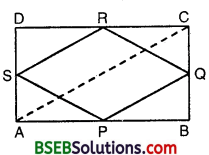Construction : Join AC.
Proof:
In ∆ ABC, P and Q; are the mid-points of sides AB and BC.
∴ PQ = AC and PQ = 12AC …(1)
Similarly, in ∆ ADC, R and S are the mid-points of sides CD and AD. .
∴ SR || AC and SR = 12AC …(2)
From (1) and (2), we get
PQ || SR and PQ = SR …(3)
Now, in quad. PQRS, its one pair of opposite sides PQ and SR is parallel and equal. [From (3)]
∴ PQRS is a parallelogram …(4)
Now, AD = BC [Opp. sides ofrect. ABCD]
⇒ 12 AD = 12 BC ⇒ AS = BQ
In ∆s APS and BPQ, we have
AP = BP [∵ P is the mid-point of AB]
∠PAS = ∠PBQ [Each = 90°]
AS = BQ [Proved above]
∴ ∆ APS ≅ ∆ BPQ [SAS Cong. axiom]
⇒ PS = PQ [C.P.C.T] … (5)
From (4) and (5), we get PQRS is a rhombus.

Question 4.
ABCD is a trapezium in which AB || DC, BD is a diagonal and E is the mid¬point of AD. A line is drawn through E parallel to AB intersecting BC at F (see figure). Show that F is the mid-point of BC.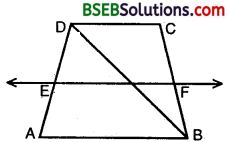Solution:
Given : In trapezium ABCD, AB || DC
E is the mid-point of AD, EF || AB.
To prove : F is the mid-point of BC.Construction : Join DB. Let it intersect EF in G.
Proof:
In A DAB, E is the mid-point of AD [Given]
EG || AB [∵ EF || AB]
∴ By converse of mid-point theorem G is the mid-point of DB.
In ∆ BCD, G is the mid-point of BD [Proved]
GF || DC [∵ AB || DC, EF || AB ⇒ DC || EF]
By converse of mid-point theorem F is the mid-point of BC.

Question 5.
In a parallelogram ABCD, E and F are the mid-points of sides AB and CD respectively (see figure). Show that the line segments AF and EC trisect the diagonal BD.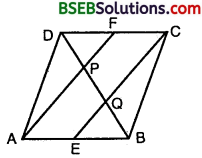Solution:
Given : E and F are the mid-points of sides AB and CD of the parallelogram ABCD whose diagonal is BD.
To prove : BQ = QP = PD
Proof:
ABCD is parallelogram [Given]
∴ AB || DC and AB = DC
[Opp. sides of parallelogram]
E is the mid-point of AB [Given]
∴ AE = 12 AB … (1)
F is the mid-point of CD
∴ CF = 12CD
⇒ CF = 12 AB [∵ CD = AB] … (2)
From (1) and (2),
AE = CF
Also AE || CF [∵ AB || DC]
Thus, a pair of opposite sides of a quadrilateral AECF are parallel and equal.
⇒ EC || AF
⇒ EQ || AP and QC || MF
In ∆ BMA, E is the mid-point of BA [Gtuen]
EQ || AP [Proved]
∴ BQ = LP
[Converse of mid-point theorem] … (3)
Similarly by taking ∆ CLD, we can prove that
DP = QP … (4)
From (3) and (4), we get
BQ = QP = PD
Hence, AF and CE trisect the diagonal AC.

Question 6.
Show that the line segments joining the mid¬points of the opposite sides of a quadrilateral bisect each other.
Solution:
Given : A quad. ABCD, P, Q, R and S are respectively the mid-points of AB, BC, CD and DA. PR and QS intersect each other at O.To prove : OP = OR,
OQ = OS.
Construction : Join PQ, QR, RS, SP, AC and BD.
Proof:
In ∆ ABC, P and Q are mid-points of AB and BC respectively.
∴ PQ || AC and PQ = 12 AC
Similarly, we can prove that
RS || AC and RS = 12AC
∴ PQ || SR and PQ = SR
Thus, a pair of opposite sides of a quadrilateral PQRS are parallel and equal.
Since the diagonals of a parallelogram bisect each other.
∴ Diagonals PR and QS of a ||gm PQRS i.e., the line segments joining the mid-points of opposite sides of quadrilateral ABCD bisect each other.

Question 7.
ABC is a triangle right angled at C. A line through the mid-point M of hypotenuse AB and parallel to BC intersects AC at D. Show that
(i) D is the mid-point of AC
(ii) MD ⊥ AC
(iii) CM = MA = 12 AB
Solution:
Given : ∆ ABC is right angled at C, M is the mid-point of hypotenuse AB. Also MD || BC.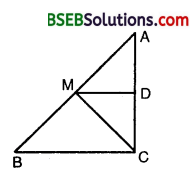To prove that :
(i) D is the mid-points of AC
(ii) MD ⊥ AC
(iii) CM = MA = 12AB.
Proof :
(i) In ∆ ABC, M is the mid-point of AB and MD || BC. Therefore, D is the mid-point of AC.

(ii) Since MD || BC. Therefore,
⇒ ∠ADM = 90° [∵ ∠ACB = 90° (given)]
But, ∠ADM + ∠CDM = 180°
[∵∠ADM and ∠CDM are angles of a linear pair]
∴ 90° + ∠CDM = 180° ⇒ ∠CDM = 90°
Thus, ∠ADM = ∠CDM = 90° … (2)
⇒ MD ⊥ AC

(iii) In ∆s AMD and CMD, we have
and, MD = MD [Common]
∴ By SAS criterion of congruence
∆ AMD ≅ ∆ CMD
⇒ MA = MC
[∵ Corresponding parts of congruent triangles are equal]
Also, MA = 12AB, since M is the mid-point of AC
Hence, CM = MA = 12AB.

## Bihar Board Class 9th Maths Chapter 8 Quadrilaterals Ex 8.2 Textbooks for Exam Preparations

Bihar Board Class 9th Maths Chapter 8 Quadrilaterals Ex 8.2 Textbook Solutions can be of great help in your Bihar Board Class 9th Maths Chapter 8 Quadrilaterals Ex 8.2 exam preparation. The BSEB STD 9th Maths Chapter 8 Quadrilaterals Ex 8.2 Textbooks study material, used with the English medium textbooks, can help you complete the entire Class 9th Maths Chapter 8 Quadrilaterals Ex 8.2 Books State Board syllabus with maximum efficiency.

## FAQs Regarding Bihar Board Class 9th Maths Chapter 8 Quadrilaterals Ex 8.2 Textbook Solutions

#### Can we get a Bihar Board Book PDF for all Classes?

Yes you can get Bihar Board Text Book PDF for all classes using the links provided in the above article.

## Important Terms

Bihar Board Class 9th Maths Chapter 8 Quadrilaterals Ex 8.2, BSEB Class 9th Maths Chapter 8 Quadrilaterals Ex 8.2 Textbooks, Bihar Board Class 9th Maths Chapter 8 Quadrilaterals Ex 8.2, Bihar Board Class 9th Maths Chapter 8 Quadrilaterals Ex 8.2 Textbook solutions, BSEB Class 9th Maths Chapter 8 Quadrilaterals Ex 8.2 Textbooks Solutions, Bihar Board STD 9th Maths Chapter 8 Quadrilaterals Ex 8.2, BSEB STD 9th Maths Chapter 8 Quadrilaterals Ex 8.2 Textbooks, Bihar Board STD 9th Maths Chapter 8 Quadrilaterals Ex 8.2, Bihar Board STD 9th Maths Chapter 8 Quadrilaterals Ex 8.2 Textbook solutions, BSEB STD 9th Maths Chapter 8 Quadrilaterals Ex 8.2 Textbooks Solutions,
Share: